﻿ Motion Analysis of In-pipe Robot Based on SMA Spring Actuator

### Motion Analysis of In-pipe Robot Based on SMA Spring Actuator

Ivan Virgala, Alexander Gmiterko, Michal KelemenOPEN ACCESSPEER-REVIEWED

## Motion Analysis of In-pipe Robot Based on SMA Spring Actuator

Ivan Virgala1,, Alexander Gmiterko1, Michal Kelemen1

1Department of Applied Mechanics and Mechatronics, Faculty of Mechanical Engineering, Technical University of Košice, Košice, Slovakia

### Abstract

The in-pipe robots play significant role for applications like inspection of pipes or wearing of cables and materials, etc. The paper deals with motion analysis of in-pipe robot, whose actuator is based on shape memory effect. At first, the principle of motion and design solution of experimental in-pipe robot is introduced. Then the mathematical model is establish by means of Newton´s mechanics. The robot using Baasic AtomPro 28-M is controlled in order to obtain autonomous of motion in the pipe. The robot can be used for pipes with circular section with diameters 10-15 mm. For real behavior determination the experiments was done and compared with mathematical model. In the conclusion the advantages and disadvantages of in-pipe robot using SMA spring – steel spring actuator are discussed.

### At a glance: Figures

1234
Prev Next

• Virgala, Ivan, Alexander Gmiterko, and Michal Kelemen. "Motion Analysis of In-pipe Robot Based on SMA Spring Actuator." Journal of Automation and Control 1.1 (2013): 21-25.
• Virgala, I. , Gmiterko, A. , & Kelemen, M. (2013). Motion Analysis of In-pipe Robot Based on SMA Spring Actuator. Journal of Automation and Control, 1(1), 21-25.
• Virgala, Ivan, Alexander Gmiterko, and Michal Kelemen. "Motion Analysis of In-pipe Robot Based on SMA Spring Actuator." Journal of Automation and Control 1, no. 1 (2013): 21-25.

 Import into BibTeX Import into EndNote Import into RefMan Import into RefWorks

### 1. Introduction

There are several kinds of areas where the pipes are used which have to be researched or explored, for example nuclear power plant, heat-exchanger, etc. Often they cannot be researched by man because of its dangerous environments and conditions or its unavailability. Hence there should be used some kind of robot which is able to moves through the pipe. In the paper the in-pipe robot will be investigated, which is for small pipe diameter designed.

There are a several issues what are under the research concerning in-pipe robots like difficult task to choose suitable actuators, sensors, power supply, etc.  There are two basic approaches for robots motion design, namely wheeled and bristled locomotion [2, 3]. Different approach is unconventional way of motion using several SMA springs creating body in square shape according to work .

In our study bristled in-pipe robot will be investigated. The bristled locomotion on the friction differences is based. In other words, coefficient of friction is lower in the forward direction in comparison with backward direction . It can be reached by suitable design solution of bristles [6, 7]. As an actuator the SMA spring in conjunction with steel spring will be used. By SMA spring dealt . Next work  dealt with inchworm robot based on SMA spring actuator. Based on mentioned works our study was motivated.

The paper is divided into following sections: At first mechanical design of in-pipe robot consisting of SMA and steel spring is introduced. Next section is dedicated to the mathematical model of robot motion in the pipe. Fourth chapter deals with experimental analysis of SMA spring. In the fifth chapter the experiment with robot is done in order to verification of locomotion with mathematical model can be done. In the conclusion the advantages and disadvantages of used in-pipe bristled robot are discussed.

### 2. Mechanical Design of In-pipe Robot

There are several issues concerning the in-pipe robots. One of them is design of their actuator. In the past there were investigated in-pipe robots with different actuators like geared DC motor, actuators based on magnetic field impact, actuators based on SMA (shape memory alloy) wires, etc. [8, 9, 10].

For our study unconventional approach was chosen by using actuator consisting of two subjects, namely SMA spring and steel spring. The actuator uses SME (shape memory effect) what means, that by heating of SMA spring, the spring is widen. In the next phase the SMA spring is cooling and now it becomes shapeable. In this phase the steel spring plays its role by shortening the SMA spring. So, by repeating of heating and cooling of SMA spring we can reach forward motion of robot. The principle of mentioned actuator in the Figure 1 is shown. The red color denotes heating phase of SMA spring and blue color denotes cooling phase of SMA spring.

During the cooling phase the SMA spring can be shortened to 16 mm. During the heating phase the SMA can be lengthened to 30 mm with over 4 N force. In software SolidWorks 3D model of in-pipe robot was designed, see Figure 2.

In-pipe robot has bristles with rectangular cross section with length 2 cm and 1 mm thickness. The bristles are from flexible material in order to adaptability to the pipe. SMA spring is from material nitinol, which has shape memory effect.

To ensuring that cooled SMA spring will moves forward not backward is achieved by bristles, attached to front and back part of robot. As will be mentioned later, expected forward motion will be reached by difference of friction between forward and backward direction of bristles.

### 3. Mathematical Model of In-pipe Robot Locomotion

The mathematical model of bristled locomotion has its foundation in the nature. The model is inspired by earthworm or inchworm, which can move by means of difference of friction causes by bristles. The sequence of motion in the Figure 3 is shown.

The locomotion is divided into two phases. The traveled distance during one locomotion sequence is δ. By repeating of these two phases the robot performs forward motion through the pipe.

3.1. First Phase of Locomotion

During the first phase the second mass moves forward because of difference of bristles friction. The first mass motion can be expressed by following equation.(1)

where Fs, Ffs and FSMA are force of steel spring, static friction and force of SMA spring, respectively. Static friction force is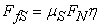(2)

where μs is static friction coefficient and FN is load force. The symbol η represents function, described by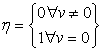(3)

The second mass motion can be expressed by following equation(4)

where Fsma, FS and Ff are force of SMA spring, force of steel spring and friction force, respectively. By consideration of dry friction between in-pipe robot bristle and wall of pipe, the friction force by Coulomb friction can be represented, according equation (5).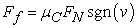(5)

where μc is Coulomb friction coefficient and FN is load force. Sgn(v) represents signum function, which can be expressed by equation (6).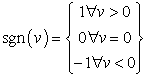(6)

Coulomb friction force depends only on mass velocity direction, not on velocity magnitude. From the equations (1) and (4) can be obtained terms for friction coefficients.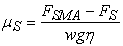(7)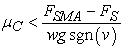(8)

where w is weight of in-pipe robot mass. It is obvious, that the higher difference between static and Coulomb friction coefficient is, the higher average velocity the robot can reaches. The difference between these two coefficients can be reached by suitable design solution of bristles.

3.2. Second Phase of Locomotion

During the second phase of locomotion, the SMA spring is cooled. When SMA spring is cooled enough it loses its force and the steel spring starts pull the first mass forward. The second phase can be described by similar way as first phase and friction coefficients are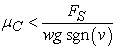(9)(10)
3.3. Average Velocity of In-pipe Robot

For average velocity determination the duration of both phases has to be expressed. Duration of the first phase from the motion equation (4) is derived by considering inertia force on right side. Duration of the second phase from the equation of motion of the first mass is derived. Duration of the first phase is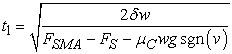(11)

Duration of second phase is(12)

Now, average velocity can be expressed by following term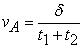(13)

Consider that force of SMA spring is in the form of step function. Then the theoretical average velocity depending on changing force of SMA spring and changing Coulomb friction coefficient can be expressed by Figure 4.

### 4. Experimental Analysis of SMA Spring and In-pipe Robot Bristles

As was mentioned above, the SMA spring changes its length by means of two actions, namely heating and cooling. The heating phase is reached by connecting of SMA spring ends to the voltage supply, what allows to current flowing through the spring, whereby the spring heats and its length expands. During the second phase the supply of current is prevented and during this time the SMA spring cools and its length is shortened by affecting of steel spring.

4.1. SMA Spring Testing

For testing of SMA spring the several steel linear springs with different stiffness were used. The stiffness of each steel spring was determined and these springs were used as opposite spring to the SMA spring in order to obtain a load for SMA spring. The voltage increased gradually with step 0.2 V up to 2 V. The results of measurements in the Figure 5 are shown.

As can be in the Figure 5 seen, the higher load force is, the higher voltage (for higher value of current) is necessary to reach full expansion of SMA spring. The load forces were obtained from steel linear springs in such way, that steel spring were connected inside of SMA spring. In other words, the steel spring pushed against SMA spring. The course of displacement is approximately linear. In the next figure, the displacement in dependence on current is shown.

The current flowing through the SMA spring is relatively high, over 4 A for 2 V. We can see that SMA spring is very demanding for current consumption what is ineligible from the view of autonomous of system, because accumulators of small dimensions for this task are inapplicable.

4.2. Friction Coefficient Measuring of In-pipe Robot Bristles

Friction coefficient of in-pipe robot bristles plays very significant role for its locomotion through the pipe. The lower Coulomb friction coefficient of bristle in comparison with static friction coefficient is, the higher velocity the robot will has. The basic assumption of robot forward motion is that the friction coefficient in forward motion in lower that friction coefficient in backward motion.

Friction coefficient in both direction by means of tribometer is determined, see Figure 7.

Using DC motor with screw the angle of inclined surface can be changed. Friction coefficient from the next equations in obtained.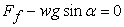(14)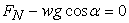(15)

By next adjustment the static friction coefficient is expressed by equation (16):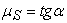(16)

Tribometer is connected to the linear potentiometer in vertical axis. The output from potentiometer through the measuring I/O card MF624 is recorded in Matlab / Simulink which cooperates with measuring card by Real Time Toolbox. The output from Simulink is measured coefficient of friction. From measuring of friction coefficient was found that(17)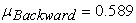(18)

Friction coefficient in forward direction is lower than friction coefficient in backward direction, whereby the forward motion is achieved. Friction coefficient in forward direction can of course be reduced by suitable mechanical modification according to user need.

### 5. Experimental Analysis of In-pipe Robot Locomotion

For experiment the glass pipe with circular section with diameter 13 mm is used. The first aim of experiment is find out the differences between the real model and mathematical model. The second aim is analysis of power consumption which is necessary to robot locomotion. The third aim of experiment is to analyze SMA spring as actuator, its advantages and disadvantages for these purposes.

In the third chapter was mentioned that one motion cycles consists of two phases. But difference between real and theoretical model is that SMA spring heats and cools the time which is significantly higher than the time which was considered in mathematical model. From the view of mathematical model the average velocity of in-pipe robot can be increased by suitable design solution of bristles what causes higher difference between static and Coulomb friction coefficient. In the reality this difference between these two coefficients is not very significant, rather negligible. This is caused because the time, necessary to heat the SMA spring and the time, necessary to cool, is very high. For example, when the current flow to the SMA spring is prevented, it takes a significant time while SMA spring cools and its force is lower in comparison with force of steel spring, which shortens SMA spring.

Connection and disconnection SMA spring to the voltage supply was automatized by means of 32-bit microcontroller Basic Atom Pro-28M. The problem with long time for cooling was partly solved by blower, which was directed to the heated place. Because of microcontroller dimensions, it could not be part of robot and it is placed out of the pipe.

Next point of experimental part is investigation of electric power consumption by actuator SMA spring – steel spring. The electric current and electric power consumption by means of MF624 were measured according to scheme, which in Matlab / Simulink was created. The example of measurement results in the Figure 9 and Figure 10 are shown.

From the Figure 9 and Figure 10 it can be seen, that first phase of motion, when SMA spring is heated, last roughly 40 seconds.

In the Figure 11 the legend shows the input voltage by which the recorded course of current was reached. As can be seen in the Figure 11, the higher current input is, the lower time the first phase lasts. In other words, by higher current we can achieved faster heating of SMA spring and then first phase duration lasts lower time. Nevertheless, the time of heating is still too high even if we use higher value of current for heating. Given results are results that were obtained using blower for faster cooling phase.

Figure 11. First phase duration caused by different value of voltage input.

The highest disadvantage of this kind of in-pipe robot is SMA spring heating and cooling phase which takes a lot of time what causes very slow locomotion, in our case only 2 mm/min.

### 6. Conclusion and Future Work

In the paper motion analysis of in-pipe robot moving in the pipe with circular section with 13 mm diameter was investigated. At first mechanical design of experimental in-pipe robot is introduced. From the mathematical model the coefficients of friction in both directions are expressed. From the mathematical model the average velocity of robot motion in the pipe is expressed but this velocity is only theoretical. The real average velocity is different because SMA spring force increases and decreases slowly.

In-pipe robot using actuator SMA spring – steel spring has advantages like very simple control, low weight, small dimensions. In spite of these advantages its disadvantages are very significant. One of the most disadvantage is the duration of heating and cooling phase. They can be a little bit modified but this modification is highly limited.

The system is autonomous in the straight pipes through the microcontroller Basic Atom Pro28-M. The next disadvantage of SMA spring is that it required high electric power consumption, whereby classical battery utilization becomes useless.

In the future the SMA spring – steel spring actuator should be modified by suitable solution of SMA spring cooling. For the tasks where does not matter on the velocity of robot and application time this kind of robot can be useful.

### Acknowledgement

The authors would like to thank to Slovak Grant Agency – project VEGA 1/1205/12 “Numerical modeling of mechatronic systems”.

### References

  Gmiterko, A., Dovica, M., Kelemen, M., Fedák, V., Mlýnková, Z., “In-pipe Bristled Micromachine”, IEEE 7th International Workshop on Advanced motion control, pp. 599-603, 2002.In article  Kelemenová, T., Kelemen, M., Miková, Ľ., Baláž, R., “Bristled In-pipe Machine Inside Pipe With Geometric Deviations”, Procedia Engineering – Elsevier / International Conference on Modeling Mechanic and Mechatronic systems, pp. 287-294, 2012.In article  Tatar, O., Mandru, D., Ardelean, I., “Development of mobile nimirobots for in pipe inspection tasks”, Mechanika, pp. 60-64, Vol. 6 (68), 2007.In article  Iwashina, Sh., Hayashi, I., Iwatsuki, N., Nakamura, K., “Development on In-Pipe Operation Micro Robots”, IEEE 5th International Symposium on Micro Machine and Human Science, pp. 41-45, 1994.In article  Wang, Z., “A Bristled-Based Pipeline Robot for I11-Constraint Pipes”, IEEE / ASME Transaction on Mechatronics, Vol. 13, No. 3, June 2008.In article  Yu, H., Ma, P., Cao, Ch., “A Novel In-Pipe Worming Robot Based on SMA”, Proceedings of the IEEE International Conference on Mechatronics & Automation, pp. 923-927, Niagara Falls, Canada, 2005.In article  Choi, H. R., Roh, S., “In-pipe Robot with Active Steering Capability for Moving Inside of Pipelines”, Bioinspiration and Robotics: Walking and Climbing Robots, pp. 375-402, Austria 2007.In article  Li, P., Ma, S., “Self-Rescue Mechanism for Screw Drive In-pipe Robots”, IEEE International Conference on Intelligent Robots and Systems, pp. 2843-2849, Taiwan 2010.In article  Yaguchi, H., Izumikawa, T., “Performance of Cableless Magnetic In-Piping Actuator Capable of High-Speed Movement by Means of Inertial Force”, Advances in Mechanical Engineering, pp. 1-9, 2001.In article  Yaguchi, H., Kamata, K., “In-piping Magnetic Actuator Capable of Inspection in a Thin Complex Pipe”, Mechanical Engineering Research, Vol. 2, No. 2, 2012.In article  Kim, S., Hawkes, E., Cho, K., Jolda, M., Foley, J., Wood, R., “Micro artificial muscle fiber using NiTi spring for soft robotics”, IEEE International Conference on Intelligent Robots and Systems, pp. 2228-2234, USA, 2009.In article PubMed  Koh, J., Cho, K., “Omega-Shaped Inchworm-Inspired Crawling Robot with Large-Index-and-Pitch (LIP) SMA Spring Actuators”, IEEE Transactions on Mechatronics, vol. 18, no. 2, 2013.In article